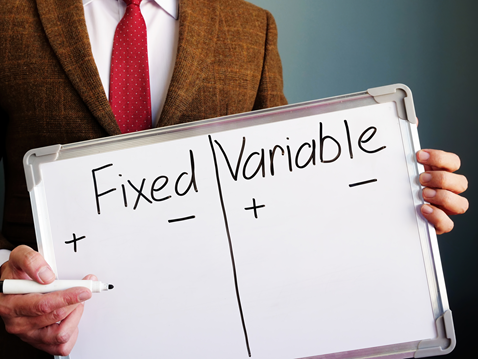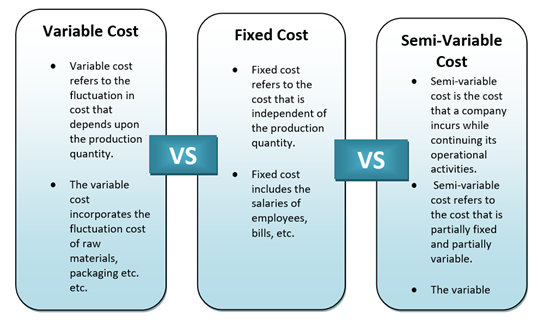# Variable Cost

• Updated on

## What is a variable cost?

Variable cost can be defined as the cost incurred by a corporate company that is proportionate to the production and sales of the company. Variable cost shows a sharp contrast with the Fixed Cost. The variable cost might constantly fluctuate depending upon the several factors involved with production and sales, such as the rise or fall in the cost of raw materials, packaging, shipping, etc. The variable cost tends to increase with the increase in the volume of production and decreases otherwise.

Summary
• Variable cost can be defined as the cost incurred by a corporate company that is proportionate to the production and sales of the company.
• Variable cost arises when the price of certain things is not specific such as the cost of raw materials, or packaging, or shipping.
• When the number of production increases, the variable cost increases. When the production quantity decreases, the variable cost decreases as well.
• The variable cost and semi-variable cost play a big role in business decision making.

## How can the concept of variable Cost be explained?

To put together in one line, the Variable Cost can be defined as the Cost depending upon production volume. Variable Cost arises when the price of certain things is not specific such as the Cost of raw materials, or packaging, or shipping. As a result of this, when the number of production increases, the variable cost increases. When the production quantity decreases, the variable Cost decreases as well. Usually, small corporate companies have a greater tendency of incurring a high variable cost.

Another sub-category of variable Cost is the partial variable Cost. Partial variable Cost can be described by the Cost, which is partly fixed and not entirely uncertain.

In contrast with Variable Cost, Fixed Cost, on the other hand, can be defined as the Cost that has zero chances of fluctuation irrespective of the production quantity.

## How can variable Cost be mathematically calculated?

Before calculating the variable Cost, always remember that it is directly proportional to the production quantity of a company. The total variable cost can be simply calculated by multiplying the total quantity of output with the Variable Cost for unit output.

Formula: Variable Cost = Total quantity of output X variable cost for unit output

## What is a Fixed Cost?

Fixed Cost can be easily defined as the Cost incurred by the company which is certain and has no chance of fluctuation. Fixed Cost acts as the opposite of Variable Cost. Fixed Costs are usually the most predictable expenditure that a company experiences every month, such as the salary of the employees, bills, debts, liabilities, etc.The total fixed Cost of a company can be simply calculated by subtracting the variable Cost from the total expenditure of a company.

Fixed cost = Total expenditure - Variable cost.

The airline companies usually have high fixed Cost as they invest in various machinery and involve a lot of labour and employees. However, companies that have high fixed Cost are hardly subject to any competition.

## How is the Variable Cost different from the Fixed Cost?

The Variable Cost incorporates the fluctuation cost of raw materials, packaging, or shipping, etc.

• Variable Cost- Variable cost refers to the fluctuation in Cost that depends upon the production quantity. The increase in the production quantity will automatically give rise to the Variable Cost. Similarly, if the output is minimised, the Variable Cost also lowers down. If production is stopped, there will be no Variable Cost in that case.• Fixed Cost-Fixed Cost refers to the Cost that is independent of the production quantity. There are a lot of expenses in a business that does not depend upon the production quantity. Such Cost includes the salaries of employees, bills, etc. Fixed Cost does not depend upon the Variable Cost. Even if there is no production, fixed Cost is something a company is bound to pay within a particular deadline.
• Semi-Variable Cost-Semi-Variable Cost is the third type of Cost a company incurs while continuing its operational activities. Semi-Variable Cost refers to the Cost that is partially fixed and partially variable.

## How does Variable Cost influence decision making?

We have already learned above that a company experiences three types of costs while executing operational activities, Fixed Cost, Variable Cost and Semi-Variable Cost. The Variable Cost and Semi-Variable Cost plays a big role in business decision making. Analysing the Fixed, Variable and Semi-Variable Cost is essential.

Semi-Variable Cost refers to such a situation when the Cost remains fixed up to a particular production limit; exceeding that limit give rise to variable Cost. Companies need to analyse that and strategically plan their production to minimise the variable Cost as much as possible.

## Examples of variable Cost

To understand the concept of Variable Cost better, let us assume a situation where the production of a packet of biscuits cost AU\$20. The cost can be broken down as AU\$8 for raw materials and AU\$12 for labour. In the following table, we have stated down how the production cost varies with the increase in the number of productions.

##### Top ASX Listed Companies

Definition:
We use cookies to ensure that we give you the best experience on our website. If you continue to use this site we will assume that you are happy with it. OK